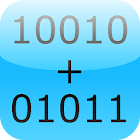Binary Calculator

• 검색 유형

모든 Android 애플리케이션 카테고리

모든 Android 게임 카테고리# Binary Calculator

by: 2.5K 8

8 사용자
평가 등급

## 스크린샷

설명

This is a free mathematical calculator, which is able to add, subtract, multiply and divide numbers in binary format (used in computers).

Any number can be represented by any sequence of bits (binary digits), they are commonly written using the symbols 0 and 1.

Best math tool for school and college! If you are a student, it will helps you to learn arithmetics for computer science and electrical engineering.

: 2.537 리뷰

"우수"

8